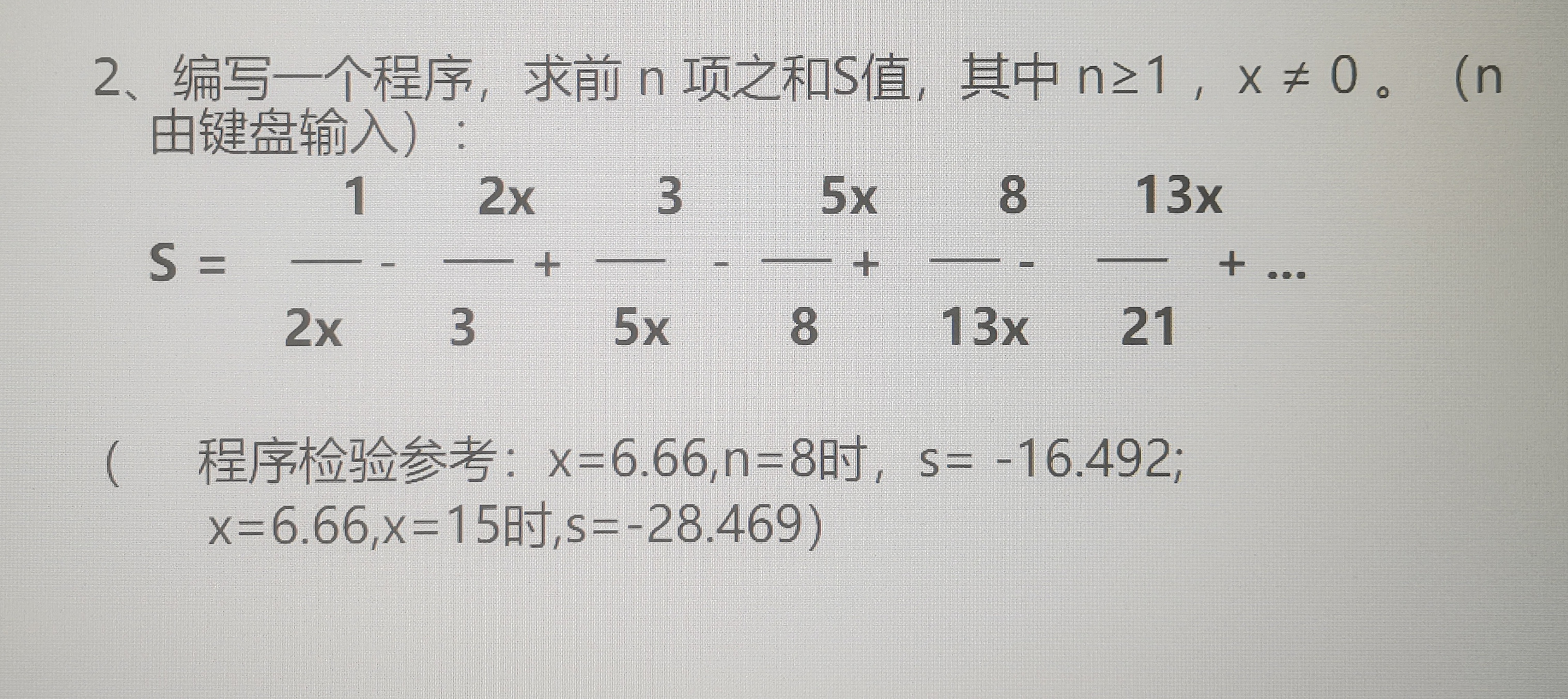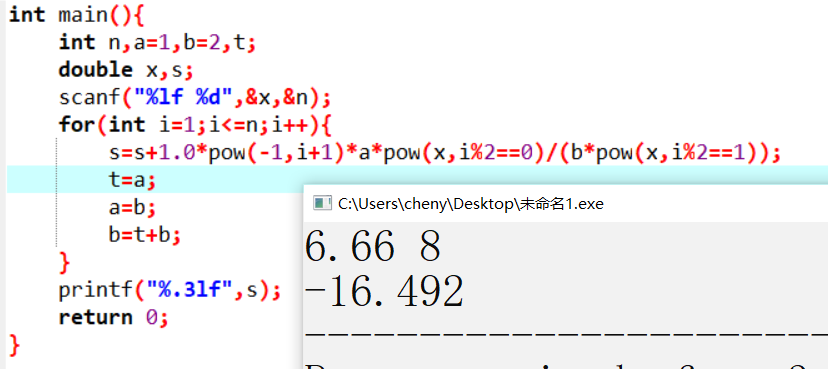2021-10-21 10:08

关于数列求和问题1/2x-2x/3+3/5x.• 写回答
• 好问题 提建议
• 追加酬金
• 关注问题
• 收藏
• 邀请回答

1条回答默认 最新

•编码图灵 2021-10-21 10:32
已采纳

分析：每一项中分子的数字部分，都是上一项分母的数字部分；
每一项中分母的数字部分，都是上一项分子与分母的数字部分之和。

#include<stdio.h>
#include<math.h>
int main(){
int n,a=1,b=2,t;
double x,s;
scanf("%lf %d",&x,&n);
for(int i=1;i<=n;i++){
//pow(-1,i+1)是为了解决第i个式子的正负号，有加有减
s=s+1.0*pow(-1,i+1)*a*pow(x,i%2==0)/(b*pow(x,i%2==1));
t=a;
a=b;
b=t+b;
}
printf("%.3lf",s);
return 0;
}觉得有用的话采纳一下哈

评论
解决 1 无用
打赏 举报